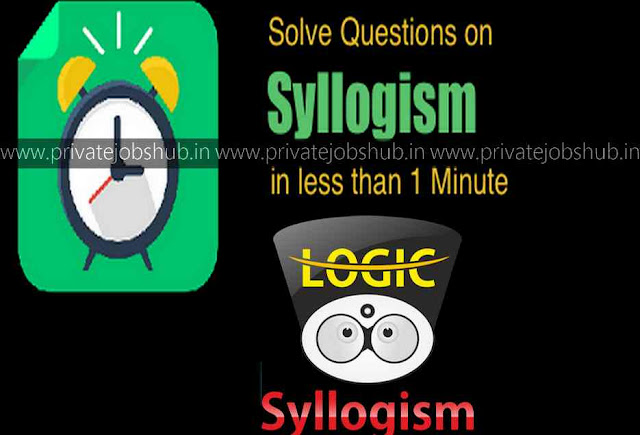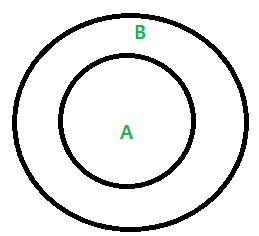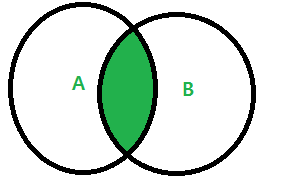## Syllogism Tricks

When we prepare for any competitive exam such as SSC or Banking exam Syllogism section cover maximum marks of weightage that’s why it is an important section to cover. But to understand Syllogism is not just that easy. So, here are providing Syllogism Tricks through which you can solve answer in 30 seconds with the help of Venn diagram. Syllogism is a verbal reasoning type problem, which is an important topic and is frequently asked in many competitive examinations in the Reasoning Section. Syllogism questions tricks will make more possibility to solve the questions with accuracy. So, let’s get started.

In Syllogism section questions contain two or more statement and these statements are followed by the number of conclusion. You have to find which conclusions logically that follows from the given statements. The best method of solving the Syllogism’s problem is through Venn Diagrams which will be discussed in this page. There are four ways through which the relationship could be made. All the shortcut tricks to solve Syllogism in easy way are described in this page. Aspirants can collect all the related information and practice the questions that are provided by the expert team members of www.privatejobshub.in

### Syllogism Tricks

SYLLOGISM Shortcut Tricks

Problem in solving questions is that it consumes a lot of time which cannot be accepted in competitive exam because competitive exam is all about time management. In a way Syllogism can make or break your final scores and sectional cutoffs and ultimately be the difference between a name in the selection list or in the registrations list for the next exam. 4-5 questions are likely to be asked from the Syllogism section in the examination. So, here we will tell you about Venn diagram through which you can solve Syllogism questions easily.

Also Practice:
Syllogism Tricks Using Venn Diagrams

We have seen so many contenders are finding difficulty in solving Syllogism questions. Usually Venn diagram method (Easy) is used to solve these but they will consume time in case of NO/Possibility conclusion cases. There is no limit as an infinite number of patterns are possible in the Syllogism Questions. Following are some of the most common patterns that are frequently asked in examination through which solving questions of Syllogism become easy.

Pattern 1: All A are B

In this type, the pattern consists of a whole circle representing A, lying within the circle representing B.Following conclusions can be deducted from the above pattern:
• Some B are A
• Some A are B
Example: All dogs are animals.

Following conclusions can be deducted from the above pattern:
• Some animals are dogs.
• Some dogs are animals.
Pattern 2: No A is B

In this type, the pattern consists of the two circles representing A and B not intersecting at all.Example: No Dog is a Cat.

Pattern 3: Some A are B

In this type, the pattern consists of the two circles representing A and B having some part in common.Following conclusions can be deducted from the above pattern:
• Some A are not B
• All A are B.
• All B are A.
• All A are B and All B are A.
Example: Some dogs are cats.

Following conclusions can be deducted from the above pattern:
• Some dogs are not cats.
• All dogs are cats.
• All cats are dogs.
• All dogs are cats and All cats are dogs.
Pattern 4: Some A are not B

In this type, the pattern consists of the circle representing A having some part which is not common with circle representing B, while the remaining portion of circle A is uncertain whether this portion touches B or not.Following conclusions can be deducted from the above pattern:
• Some A are B.
• No A is B.
Rules to Solve “SYLLOGISM” Questions

Solving Syllogism is like a fun game if you understand the concepts. Basics must be clear to get fun in Solving Syllogism questions. Follow the tips given below to solve question based on Syllogism. First and foremost have a quick glance at the Main rules to solve Syllogism Problems:

 All + All All All + No No All + Some No Conclusion Some + All Some Some + No Some Not Some + Some No Conclusion

If the conclusion provided in the question is in “Possibility” case then you must proceed as per the following rules.

 If All A are B Some B are Not A is a Possibility. If Some B are Not A All A are B is a Possibility. If Some A are B All A are B is a Possibility & All B are A is a Possibility.

In possibilities cases, we have to create all possibilities to find whether the given conclusion is possible or not. If it is possible and satisfies the given statement than given conclusion will follow otherwise conclusion will not follow.

Practice:
Syllogism Tips and Tricks

Following are some tips and tricks to solve syllogism through venn diagram. If you follow the criteria, possibility of accurate answer will be more and in future daily practice of these questions will make you perfect and you can solve Syllogism question in 10 seconds.
• The first step is to make a Venn diagram.
• Go through all the statements given in the question one by one and make venn diagram with the help of statements.
• Understand how to draw Venn Diagrams for each statement which will be possible through practice.
• Try to observe if, there are any familiar concepts or statement in the given syllogism.
• After this try to find out the conclusion of Syllogism.
• Understand how to draw a conclusion for each concept/statement.
• There is no need to memorize any statement or conclusion.
• All you need is to understand the Concept and draw the venn diagram accordingly.
• The key point here is to attempt the question in a sequential manner.
Final Note:

Hope this page will proof useful to you and you can solve Syllogism question with more confident and accuracy. Syllogism Tricks to solve questions quickly will give you positive result and you will get expected cutoff marks also. Join us on Facebook or Google + or bookmark our web page that is www.privatejobshub.in to get latest and instant updates.